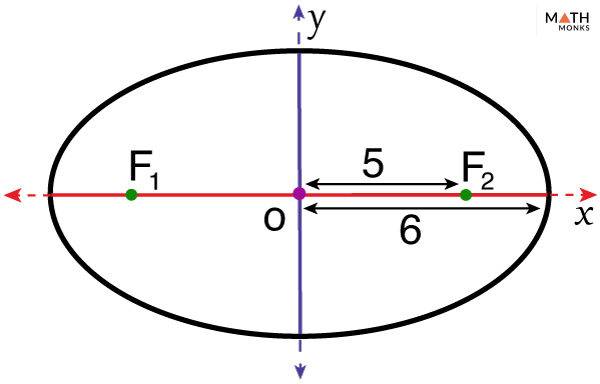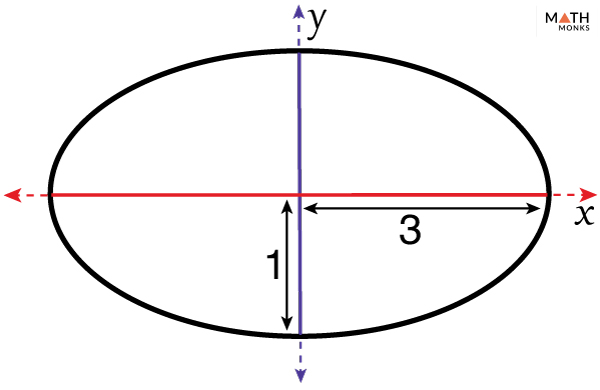# Ellipse

## Definition

An ellipse is a closed curved plane formed by a point moving so that the sum of its distance from the two fixed or focal points is always constant. It is formed around two focal points, and these points act as its collective center.

We can also define ellipse as the shape formed when a straight plane intersects a right circular cone at an angle greater than zero degrees.

The flatness or roundness of an ellipse is determined by a property termed eccentricity. It is represented by ‘e’.

## Parts

• Focus: Has 2 foci. The foci (singular: focus) always lie on the major axis.
• Center: The midpoint between the 2 foci. The major and minor axes intersect at this point at 90°.
• Major axis: It is the distance between the end vertices. Center divides the major axis into 2 equal halves. Each half is called semi-major axis or major radius, represented by ‘a’.
• Minor axis: It is the distance between the end co-vertices. Center divides the minor axis into 2 equal halves. Each half is called semi-minor axis or minor radius, represented by ‘b’.
• Vertex: It is the point where the ellipse intersects the major axis. In other words, the 2 extreme points that form the major axis are the vertices.
• Co-vertex: It is the point where the ellipse intersects the minor axis. In other words, the 2 extreme points that form the minor axis are the co-vertices.

Ellipses can have only two orientations based on planes (x-axis or y-axis) the foci lie on.

Horizontal ellipse

Ellipse having the major axis on the x-axis.

Vertical Ellipse

Ellipse having the major axis on the y-axis.

## Properties

1. Ellipses have two foci or focal points; here F1 & F2 are the 2 foci.
2. An ellipse has a center, and two axes — major axis and minor axis; here center is at (0,0), AB is major axis, CD is minor axis.
3. The sum of the distances between any point (P) on the ellipse and the two focal points is constant, and it is equal to the total length of the major axis; here PF1+PF2 = 2a is constant.
4. The eccentricity value of all ellipses is always less than one (e < 1)

## Eccentricity of Ellipse

The eccentricity of an ellipse measures how flat or round an ellipse is.

The more flat an ellipse is, the greater is its eccentricity, and the more round it is, its eccentricity is closer to zero. Eccentricity as 1 is a straight line, and zero will be a perfect circle. It is less than 1.  (e<1).

The formula for the eccentricity of an ellipse is given below:

### How To Find The Eccentricity Of An Ellipse

Here we will derive the above formula of the eccentricity of the ellipse.

O is the center at the origin. F1 (-c,0) and F2 (c,0) are the foci.

A & B are the vertices. AB = major axis = 2a

As we know,

c= 1/2 distance between the 2 focal points, and a= semi-major axis

P (0,b) is a point on ellipse.

Let PO=b=semi-minor axis, PF1 + PF2 = 2a, OF2=c,

As we know, ∠POB=∠POA=90°, therefore, ∠POF2 = 90°

Applying Pythagoras theorem for right-angled △POF2,

∴ PF2 =√(b2 + c2), and also PF2 = a

∴ c= √(a2-b2)

Putting the value of c in the formula of eccentricity, we get,

e = √(1-b2/a2)

= √{( a2-b2)/a2}

= √(c2/a2) =c/a

∴ e=c/a

Thus we get the standard simplified formula of the eccentricity of the ellipse.

Note: If a=b, (like in the case of a circle)

then c =√(a2-b2) = 0

∴ e = 0 (eccentricity of circle is zero)

∴ 0≤e>1

Let us solve an example involving the eccentricity of the ellipse.Find the eccentricity of an ellipse with a major axis 12 and the distance between the focus and center is 5.

Solution:

As we know,
Eccentricity (e) = c/a, here, c = 5 units, a = 6 units
= 5/6 = 0.833 units

## Equation

The standard form of equation of an ellipse is x2/a2 + y2/b2 = 1, where a = semi-major axis, b = semi-minor axis.

Let us derive the standard equation of an ellipse centered at the origin

### Derivation

The equation of ellipse focuses on deriving the relationships between the semi-major axis, semi-minor axis, and the focus-center distance.

Our aim is to find the relationships of a, b, and c.

Length of major axis = 2a.

Length of minor axis = 2b.

Distance between the foci = 2c.

Take another point Q on one end of the minor axis.

Find the sum of the distances of Q from foci F1 and F2

QF1+ QF2 = √(b2+c2)+ √(b2+c2)

QF1+ QF2 = 2 [√(b2+c2)]

As Q lie on the ellipse. As per the definition, sum of distances between any point on ellipse with the foci is constant. (2a according to the diagrams)

2√(b2+c2)= 2a

√(b2+c2)= a

Squaring both sides:

b2 + c2 = a2

c2 = a2 – b2

• Now consider any point S on the same ellipse.
• Find out the sum of its distances from the two foci F1 and F2, which is 2a.
• The above steps prove that the sum of the distances of any point on the ellipse from the foci is 2a

SF1 + SF2 = 2a

Now applying the distance formula, we get

=>√(x+c)2+y2 + √(x−c)2+y2 = 2a

√[(x+c)2+y2]= 2a – √[(x−c)2+y2]

Squaring both sides

(x + c)2 + y2 = 4a2 + (x – c)2 + y2 – 4a√(x−c)2+y2

x2 + c2 + 2cx + y2

= 4a2 + x2 + c2 – 2cx + y2 – 4a√[(x−c)2+y2]

4cx – 4a2 = – 4a√[(x−c)2+y2]

a2 – cx = a√[(x−c)2+y2]

Squaring both sides and simplifying, we have

x2/a2+y2 /(c2−a2)=1

As we have c2 = a2 – b2 we can substitute this in the above equation.

We can substitute this in the above equation

x2/a2+y2/b2=1

This is the standard equation of an ellipse centered at the origin.

### Writing Equations of Ellipses in Standard Form

Here, P (x,y) is a point on the ellipse, major axis = 2a, minor axis = 2b

Let us solve few examples involving equations of an ellipse

Find the equation of the ellipse with a major axis on the X-axis and having coordinates (2, -2) and (-1, 3)

Solution:

As we know, the standard equation of an ellipse is given by
x2 /a2+y2/b2 = 1, here, points (2, 2) and (-1, 3) lie on the ellipse,
Now, there can be two possible situations:
Case 1: (2)2/a2 + (2)2/b2 =1, when it has coordinates (2, 2)
Case 2:  (-1)2/a2 + (3)2/b2 =1, when it has coordinates (-1, 3)
Comparing with the standard equation, we get
a2 = 35/8
b2 =35/3
Putting values of a and b on the equation of an ellipse, we get
x2 ÷ (35/8) + y2 ÷ (35/3) =1
8x2 + 3y2=35

Find the values of a (major axis) and b (minor axis) from the equation 4x2 + 25y2 =100.

Solution:

4x2 + 25y2 =100 (Given)
Dividing by 100 at both sides
=> x2/25 + y2 /4 = 1
Comparing this equation with equation of an ellipse,  x2/a2 + y2 /b2=1
=>a2 = 25 => a=5
=> b2 = 4 => b=2
Thus the value of the major axis (2a) = 10 and minor axis (2b) = 4

## Formulas

### Area

The formula to find the area of an ellipse is given below:

Now, let us solve a problem involving the area of an ellipse to clear your concept better.Find the area of an ellipse whose major axis is 6 units and the minor axis is 2 units in length.

Solution:

Area (A) = πab, here a = 3, b = 1, π = 3.141 = 22/7
= π x 3 x 1
= π x3
= 9.424 sq units
Thus the area of the ellipse is 9.424 sq units

We generally consider the half of any two-dimensional shape when talking about the area. We already have found out the area of an ellipse in the above example. Now let’s talk about the half of an ellipse.

Semi-ellipse is one-half of an ellipse. When an ellipse is cut, it forms a curved side (arc) and a straight side. The straight side is called the height or Rise. It is denoted as H of the semi-ellipse.

Since the area of an ellipse is πab.

Therefore,

The formula of the area of a semi-ellipse is 1/2(πab) sq. units

Let us solve an example.Find the area of a semi-ellipse whose major axis is 8 units and the minor axis is 2 units in length.

Solution:

As we know,
Area (A) = 1/2(πab), here a = 4, b = 1
= 1/2(π x 4 x 1) sq. units
= 6.283 sq. units.

### Perimeter

The formula to find the perimeter of an ellipse is given below:

Now, let us solve a problem involving the perimeter of an ellipse to clear your concept better.

Find the perimeter of an ellipse whose major axis is 6 units and the minor axis is 2 units in length.

Solution:

Perimeter (P) = 2π x √(a2 + b2)/2, here a = 3, b = 1, π = 3.141 = 22/7
=2π [√(32 + 12)/2)
= 2π√5
=14.049 units
Thus the perimeter of the ellipse is 14.049 units

## Examples in Real Life

The two-dimensional shape of a rugby ball.

Whispering galleries. Two people standing under a semi-elliptical roof can whisper and communicate with each other. Grand Central Terminal in New York is one of the real examples of such galleries.

Lithotripter, a medical device, applies the principle of an ellipse. It helps to break the stones in kidneys by shockwaves applied from one focal point in the ellipsoidal reflector in the device, whereas; the kidney is placed at the other focal point.

The orbital movement of the planets in our solar system is also in an elliptical pattern.

• More Resources